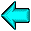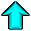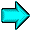# 7. Device Color Characterization

The X protocol provides explicit RGB values, which are used to directly drive a monitor, and color names. RGB values provide a mechanism for accessing the full capabilities of the display device, but at the expense of having the color perceived by the user remain unknowable through the protocol. Color names were originally designed to provide access to a device-independent color database by having the server vendor tune the definitions of the colors in that textual database. Unfortunately, this still does not provide the client any way of using an existing device-independent color, nor for the client to get device-independent color information back about colors that it has selected.

Furthermore, the client must be able to discover which set of colors are displayable by the device (the device gamut), both to allow colors to be intelligently modified to fit within the device capabilities (gamut compression) and to enable the user interface to display a representation of the reachable color space to the user (gamut display).

Therefore, a system is needed that will provide full access to device-independent color spaces for X clients. This system should use a standard mechanism for naming the colors, be able to provide names for existing colors, and provide means by which unreachable colors can be modified to fall within the device gamut.

We are fortunate in this area to have a seminal work, the 1931 CIE color standard, which is nearly universally agreed upon as adequate for describing colors on CRT devices. This standard uses a tri-stimulus model called CIE XYZ in which each perceivable color is specified as a triplet of numbers. Other appropriate device-independent color models do exist, but most of them are directly traceable back to this original work.

X device color characterization provides device-independent color spaces to X clients. It does this by providing the barest possible amount of information to the client that allows the client to construct a mapping between CIE XYZ and the regular X RGB color descriptions.

Device color characterization is defined by the name and contents of two window properties that, together, permit converting between CIE XYZ space and linear RGB device space (such as standard CRTs). Linear RGB devices require just two pieces of information to completely characterize them:

• A 3 × 3 matrix M and its inverse M-1, which convert between XYZ and RGB intensity (RGBintensity):

RGBintensity = M × XYZ
XYZ = M-1 × RGBintensity

• A way of mapping between RGB intensity and RGB protocol value. XDCCC supports three mechanisms which will be outlined below.
If other device types are eventually necessary, additional properties will be required to describe them.

## 7.1. XYZ <-> RGB Conversion Matrices

Because of the limited dynamic range of both XYZ and RGB intensity, these matrices will be encoded using a fixed-point representation of a 32-bit two's complement number scaled by 227, giving a range of -16 to 16 - ε, where ε = 2 -27.

These matrices will be packed into an 18-element list of 32-bit values, XYZ -> RGB matrix first, in row major order and stored in the XDCCC_LINEAR_RGB_MATRICES properties (format = 32) on the root window of each screen, using values appropriate for that screen.

This will be encoded as shown in the following table:
XDCCC_LINEAR_RGB_MATRICES property contents

M0,0 INT32 Interpreted as a fixed-point number -16 <= x < 16
M0,1 INT32
...
M3,3 INT32
M-10,0 INT32
M-10,1 INT32
...
M-13,3 INT32

## 7.2. Intensity <-> RGB Value Conversion

XDCCC provides two representations for describing the conversion between RGB intensity and the actual X protocol RGB values:
1. RGB value/RGB intensity level pairs
2. RGB intensity ramp

In both cases, the relevant data will be stored in the XDCCC_LINEAR_RGB_CORRECTION properties on the root window of each screen, using values appropriate for that screen, in whatever format provides adequate resolution. Each property can consist of multiple entries concatenated together, if different visuals for the screen require different conversion data. An entry with a VisualID of 0 specifies data for all visuals of the screen that are not otherwise explicitly listed.

The first representation is an array of RGB value/intensity level pairs, with the RGB values in strictly increasing order. When converting, the client must linearly interpolate between adjacent entries in the table to compute the desired value. This allows the server to perform gamma correction itself and encode that fact in a short two-element correction table. The intensity will be encoded as an unsigned number to be interpreted as a value between 0 and 1 (inclusive). The precision of this value will depend on the format of the property in which it is stored (8, 16 or 32 bits). For 16-bit and 32-bit formats, the RGB value will simply be the value stored in the property. When stored in 8-bit format, the RGB value can be computed from the value in the property by:
 RGBvalue = Property Value × 65535 255

Because the three electron guns in the device may not be exactly alike in response characteristics, it is necessary to allow for three separate tables, one each for red, green, and blue. Therefore, each table will be preceded by the number of entries in that table, and the set of tables will be preceded by the number of tables. When three tables are provided, they will be in red, green, blue order.

This will be encoded as shown in the following table:
XDCCC_LINEAR_RGB_CORRECTION Property Contents for Type 0 Correction

VisualID0 CARD Most-significant portion of VisualID
VisualID1 CARD Exists if and only if the property format is 8
VisualID2 CARD Exists if and only if the property format is 8
VisualID3 CARD Least-significant portion, exists if and only if the property format is 8 or 16
type CARD 0 for this type of correction
count CARD Number of tables following (either 1 or 3)
length CARD Number of pairs &endash; 1 following in this table
value CARD X Protocol RGB value
intensity CARD Interpret as a number 0 <= intensity <= 1
... ... Total of length+1 pairs of value/intensity values
lengthg CARD Number of pairs &endash; 1 following in this table (if and only if count is 3)
value CARD X Protocol RGB value
intensity CARD Interpret as a number 0 <= intensity <= 1
... ... Total of lengthg+1 pairs of value/intensity values
lengthb CARD Number of pairs &endash; 1 following in this table (if and only if count is 3)
value CARD X Protocol RGB value
intensity CARD Interpret as a number 0 <= intensity <= 1
... ... Total of lengthb+1 pairs of value/intensity values

The VisualID is stored in 4, 2, or 1 pieces, depending on whether the property format is 8, 16, or 32, respectively. The VisualID is always stored most-significant piece first. Note that the length fields are stored as one less than the actual length, so 256 entries can be stored in format 8.

The second representation is a simple array of intensities for a linear subset of RGB values. The expected size of this table is the bits-per-rgb-value of the screen, but it can be any length. This is similar to the first mechanism, except that the RGB value numbers are implicitly defined by the index in the array (indices start at 0):
 RGBvalue = Array Index × 65535 Array Size - 1
When converting, the client may linearly interpolate between entries in this table. The intensity values will be encoded just as in the first representation.

This will be encoded as shown in the following table:
XDCCC_LINEAR_RGB_CORRECTION Property Contents for Type 1 Correction

VisualID0 CARD Most-significant portion of VisualID
VisualID1 CARD Exists if and only if the property format is 8
VisualID2 CARD Exists if and only if the property format is 8
VisualID3 CARD Least-significant portion, exists if and only if the property format is 8 or 16
type CARD 1 for this type of correction
count CARD Number of tables following (either 1 or 3)
length CARD Number of elements &endash; 1 following in this table
intensity CARD Interpret as a number 0 <= intensity <= 1
... ... Total of length+1 intensity elements
lengthg CARD Number of elements &endash; 1 following in this table (if and only if count is 3)
intensity CARD Interpret as a number 0 <= intensity <= 1
... ... Total of lengthg+1 intensity elements
lengthb CARD Number of elements &endash; 1 following in this table (if and only if count is 3)
intensity CARD Interpret as a number 0 <= intensity <= 1
... ... Total of lengthb+1 intensity elementsChristophe Tronche, ch@tronche.com